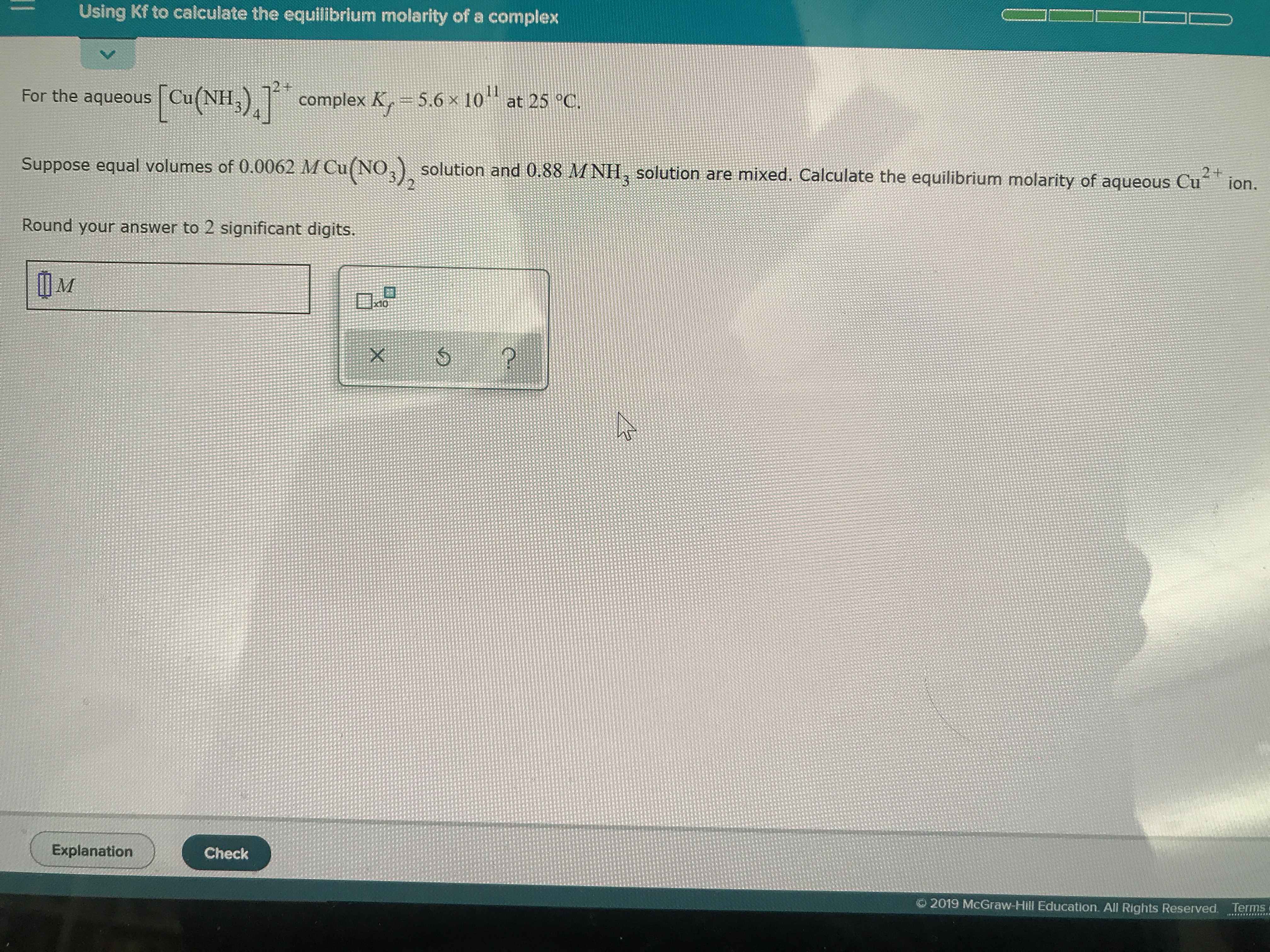# For the aqueous [Cu(NH3)4]^2+ complex Kf = 5.6 x 10^11 at 25 °C. Suppose equal volumes of 0.0062 M Cu(NO3)2 solution and 0.88 M NH3 solution are mixed. Calculate the equilibrium molarity of aqueous Cu^2+ ion. Round our answer to 2 significant digits.